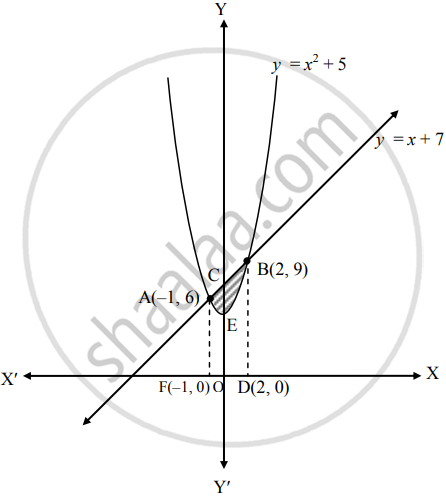# Find the area of the region included between y = x2 + 5 and the line y = x + 7 - Mathematics and Statistics

Sum

Find the area of the region included between y = x2 + 5 and the line y = x + 7

#### Solution

Given equation of the curve is

y = x2 + 5   ......(i)

and equation of the line is

y = x  7     ......(ii)

Find the points of intersection of y = x2 + 5 and y = x + 7

Substituting (ii) in (i), we get

x + 7 = x2 + 5

∴ x2 – x – 2 = 0

∴ (x – 2)(x + 1) = 0

∴ x = 2 or x = –1

When x = 2, y = 9 and when x = –1, y = 6

∴ The points of intersection are B(2, 9) and A(–1 , 6).

Draw BD ⊥ OX.Required area = area of the region AEBCA

= area of the region FODBCAF − area of the region FODBEAF

= area under the line y = x + 7 – area under the curve y = x2 + 5

= int_(-1)^2 (x + 7)  "d"x - int_(-1)^2 (x^2 + 5)  "d"x   .....[From (ii) and (i)]

= int_(-1)^2 (x + 7 - x^2 - 5)  "d"x

= int_(-1)^2 (x - x^2 + 2)  "d"x

= [x^2/2]_(-1)^2 - [x^3/3]_(-1)^2  + 2[x]_(-1)^2

= 1/2[2^2 - (-1)^2] - 1/3[2^3 - (-1)^3] + 2[2 - (-1)]

= 3/2 - 9/3 + 6

= 9/2 sq.units

Concept: Area Between Two Curves
Is there an error in this question or solution?